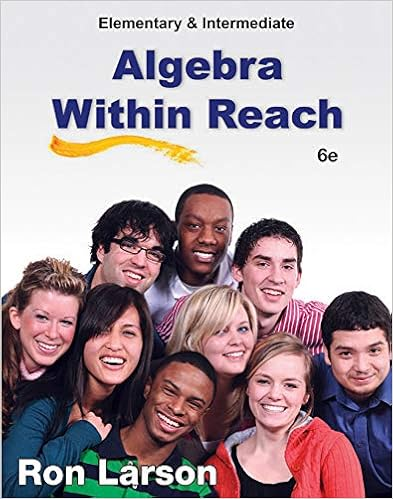# For find the value of c that makes the expression a

• Test Prep
• 4

This preview shows page 3 - 4 out of 4 pages.

##### We have textbook solutions for you!
The document you are viewing contains questions related to this textbook.The document you are viewing contains questions related to this textbook.
Chapter 10 / Exercise 59
Elementary and Intermediate Algebra: Algebra Within Reach
LarsonExpert Verified
For questions 20 - 21, find the value of c that makes the expression a perfect square trinomial. Then write the expression as the square of a binomial. 20. x 2 21. x 2    For questions 22 - 29, choose the best answer and place the corresponding letter in the blank. _____ 22. What is the vertex of the graph of y       ? A. 3, 4 ( ) B. 3, 4 C. 3,    D. 3,  4 ( )
_____ 24. If b 2      , then the type and number of zeros for the quadratic equation are which of the following?
_____23.If ax 2  is a perfect square trinomial, then the graph of y   has exactly how many x-intercepts?
##### We have textbook solutions for you!
The document you are viewing contains questions related to this textbook.The document you are viewing contains questions related to this textbook.
Chapter 10 / Exercise 59
Elementary and Intermediate Algebra: Algebra Within Reach
LarsonExpert Verified
_____ 25. Which of the following is equivalent to i 41 ?
_____ 26. What is the conjugate of 4   ? A. 3   B. 3 4 i C. 4   D. 5
_____ 27. Which of the following quadratic equations is in vertex form?
_____ 28. Which of the following is a perfect square trinomial?
_____ 29. In which direction does the parabola y       open?
30. Derive the Quadratic Formula from y   .
•••